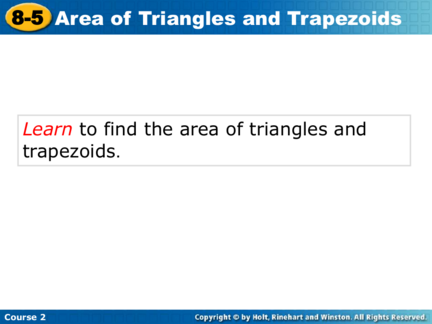# Area of Triangles and TrapezoidsContributed by:This pdf briefly explains finding the area of triangles and trapezoids. The area A of a triangle is half the product of its base b and its height h. A parallelogram can be divided into two congruent trapezoids. The area of each trapezoid is one-half the area of the parallelogram.
1. Areaof
8-5 Area ofTriangles
Trianglesand
andTrapezoids
Trapezoids
Learn to find the area of triangles and
trapezoids.
Course 2
2. 8-5 Area of Triangles and Trapezoids
A diagonal of a parallelogram divides the
parallelogram into two congruent triangles. So the
area of each triangle is half the area of the
parallelogram.
Height
Height
Base Base
The base of a triangle can be any side. The height of
a triangle is the perpendicular distance from the
base to the opposite vertex.
Course 2
3. 8-5 Area of Triangles and Trapezoids
AREA OF A TRIANGLE
The area A of a triangle
is half the product of its A = 1 bh h
2
base b and its height h.
b
Course 2
4. 8-5 Areas of Triangles and Trapezoids
Additional Example 1A: Finding the Area of a
Triangle
Find the area of the triangle.
A.
A = 1 bh Use the formula.
2
5
A = 1 (8 · 5) Substitute 8 for b and
8 2 5 for h.
A = 20
The area of the triangle is 20 square units.
Course 2
5. 8-5 Area of Triangles and Trapezoids
Additional Example 1B: Finding the area of a
Triangle
Find the area of the triangle.
B.
A = 1 bh Use the formula.
2
12
A =1 (9 · 12) Substitute 9 for b and
2 12 for h.
9
A = 54
The area of the triangle is 54
square units.
Course 2
6. 8-5 Areas of Triangles and Trapezoids
Try This: Example 1A
Find the area of the triangle.
A.
A = 1 bh Use the formula.
2
9 A = 1 (6 · 9) Substitute 6 for b and
2 9 for h.
6 A = 27
The area of the triangle is 27 square units.
Course 2
7. 8-5 Areas of Triangles and Trapezoids
Try This: Example 1B
Find the area of the triangle.
B.
A = 1 bh Use the formula.
2
10 A = 1 (7 · 10) Substitute 7 for b and
2 10 for h.
A = 35
7
The area of the triangle is 35 square units.
Course 2
8. 8-5 Area of Triangles and Trapezoids
A parallelogram can be divided into two
congruent trapezoids. The area of each trapezoid
is one-half the area of the parallelogram.
Area of a trapezoid = 1 (base of
2
parallelogram)(height).
Course 2
9. 8-5 Area of Triangles and Trapezoids
The two parallel sides of a trapezoid are its bases.
If we call the longer side b1 and the shorter side
b2, then the base of the parallelogram is b1 + b2.
Area of a trapezoid = 1 (base 1 +
2
base 2)(height).
Course 2
10. 8-5 Area of Triangles and Trapezoids
AREA OF A TRAPEZOID
The area of a trapezoid b2
is half its height A = 1 h(b1 + b2)
multiplied by the sum of 2 h
its two bases. b1
Course 2
11. 8-5 Areas of Triangles and Trapezoids
Additional Example 2A: Finding the Area of a
Trapezoid
Find the area of the trapezoid.
A. 5 in. A = 1 h(b1 + b2) Use the formula.
2
6 in. A = 1 · 6(5 + 9) Substitute.
2
A = 1 · 6(14) Add.
9 in. 2
A = 42 Multiply.
The area of the trapezoid is 42 in2.
Course 2
12. 8-5 Areas of Triangles and Trapezoids
Additional Example 2B: Finding the Area of a
Trapezoid
Find the area of the trapezoid.
B.
12 cm A = 1 h(b1 + b2) Use the formula.
2
7 cm A = 1 · 7(12 + 16) Substitute.
2
16 cm A = 1 · 7(28) Add.
2
A = 98 Multiply.
The area of the trapezoid is 98 cm2.
Course 2
13. 8-5 Areas of Triangles and Trapezoids
Try This: Example 2A
Find the area of the trapezoid.
A.
A = 1 h(b1 + b2) Use the formula.
2
A = 1 · 6(11 + 4) Substitute.
11 in. 4 in. 2
6 in.
A = 1 · 6(15) Add.
2
A = 45 Multiply.
The area of the trapezoid is 45 in2.
Course 2
14. 8-5 Areas of Triangles and Trapezoids
Try This: Example 2B
Find the area of the trapezoid.
B.
A = 1 h(b1 + b2) Use the formula.
16 cm 2
A = 1 · 9(5 + 16) Substitute.
9 cm 2
5 cm A = 1 · 9(21) Add.
2
A = 94.5 Multiply.
The area of the trapezoid is 94.5 cm2.
Course 2
15. 8-5 Area
Insert
ofLesson
Triangles
Title
and
Here
Trapezoids
Lesson Quiz
Find the area of each figure.
1. 2. 9 in2
45 ft2 3 in.
9 ft
10 ft 6 in.
8 ft 10 ft
3. 4. 87.5 ft2
6 ft 60 ft2 7 ft
12 ft 15 ft
5. What is the height of a triangle with area
36 cm2 and a base 9 cm? 8 cm
Course 2
16. Homework
8-5 Worksheet
Study for fraction quiz Thursday